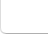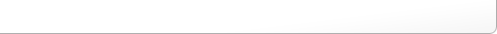## Hypoperfusion Asymmetry Index

The Hypoperfusion Asymmetry Index (HAI) measures the volume of significant hypoperfusion voxels based on p-values and extent thresholds in each hemisphere of the brain. This is a powerful tool for lateralizing seizure onset to the correct hemisphere for post-ictal injections.

### Calculating the HAI

In order to calculate the hypoperfusion asymmetry index follow the steps below:

2. At the results step of your hypoperfusion contrast, click on the small volume correction button (SVC).
3. For the search volume, choose image. Then choose the binary image Left_Hemi.img.
4. Add up the number of significant voxels in the left hemisphere (shown in your statatistics table under cluster volume, k).
5. Repeat, using Right_Hemi.img to calculate the number of significant hypoperfusion voxels in the right hemisphere.
6. HAI = ((kleft  kright) /(kleft + kright))

### Interpreting HAI

A positive HAI indicates more hypoperfusion in the left hemisphere, while a negative HAI indicates more hypoperfusion in the right hemisphere.

Small positive or negative values of magnitude less than ~0.10 for the HAI may not indicate a significant asymmetry. (see McNally et al., 2005 for details of interpretation).

NOTE:The data for the sample analysis represents a patient with an ictal injection. Therefore, localization should be based on CBF increases using the "Reading Rules" and calculating the HAI for this patient is not expected to correctly lateralize the hemisphere of seizure onset.
For purposes of reviewing the calculations, let us pretend that the sample patient data were from a post-ictal injection. The HAI would then be calculated as follows:
HAI = kleft  kright) / (kleft + kright)
HAI = (5658  965) /(5658 + 965) (based on k values as described above)
HAI = 0.71
Since the HAI is positive, this would lateralize seizure onset to the left hemisphere. Based on McNally et al., 2005, this lateralization is expected to be correct in approximately 80% of patients if the injection occurred in the post-ictal period (which it did not for this patient).